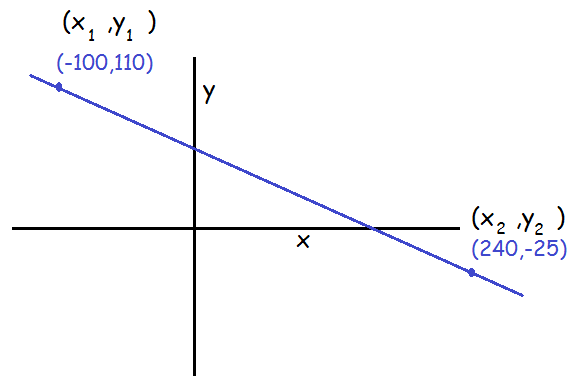# Tutoring math, you know this skill is essential.  The math tutor provides a quick explanation and example.

The concept of slope is known to all in everyday life.  In math, it is defined as follows:

slope=rise/run=m=(y2-y1)/(x2-x1)

Indeed, slope is often referred to as m. The rise refers to the change in height; the run, to the change in horizontal position.

This article assumes you understand points on the cartesian plane. If you don’t, see my article here.

Example: Let’s imagine you need the slope of the line pictured here:We need to realize three facts:

1) Every point is (x,y); x means horizontal position, while y means vertical.

2) “Change” means the final value minus the initial value.

3) Once again, the rise refers to the change in height; the run, to the change in horizontal position.

Now we involve the equation for slope:

m=(y2-y1)/(x2-x1)

Notice that (x1,y1) means “the first point”, while (x2,y2) means “the second point.” It’s helpful to label your two points (x1,y1) and (x2,y2). Next, carefully insert the values in their right places in the equation:

m=(-25-110)/(240-(-100))

Simplifying, we get

m=-135/340=-27/68 or -0.397

Slope is always pictured from left to right. If a line rises to the right, its slope is positive. The line in our example falls to the right; hence, its slope is negative.

The slope of a line has many applications, which I’ll be discussing in future posts:)

Jack of Oracle Tutoring by Jack and Diane, Campbell River, BC.

Tagged with: ,